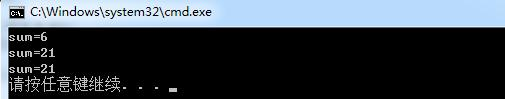# 静态成员变量和静态成员函数在C++类中的作用

• 静态全局变量，在其他文件中可以定义相同的名字而不会发生冲突；
• 静态局部变量保存在全局数据区，而不是保存在栈中，每次的值保持到一下次的调用，直到下次赋值；
• 静态函数只能在声明它的文件当中可见，不能被其他文件使用，在其他文件中可以定义相同名字的函数，不会发生冲突。

1、概念

2、静态数据成员初始化

<数据类型><类名>::<静态数据成员> = <值>;
eg:int MyClass::sum = 0;

1、概述

2、普通成员函数和静态成员函数的区别

3、举例说明

#include <iostream>
using namespace std;

class MyClass {
public:
MyClass(int a,int b,int c);
static void func();
private:
int a, b, c;
static int sum;
};
int MyClass::sum = 0;

MyClass::MyClass(int a,int b,int c)
{
this->a = a;
this->b = b;
this->c = c;
sum += a + b + c;
}

void MyClass::func()
{
cout << "sum=" << sum << endl;
}

void main()
{
MyClass M(1, 2, 3);
M.func();

MyClass N(4,5,6);
N.func();

MyClass::func();// 静态成员函数的访问

return;
}©️2019 CSDN 皮肤主题: 大白 设计师: CSDN官方博客